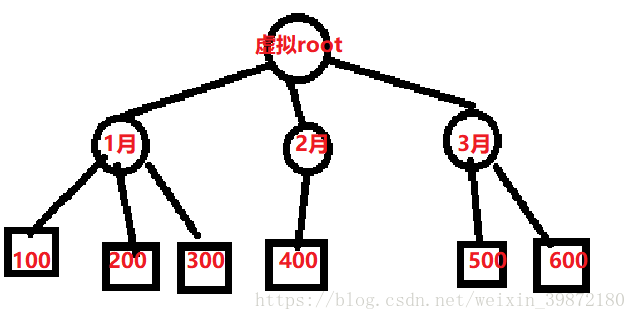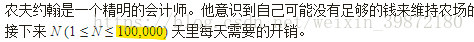OJ 8209LittleBlack 1月 27, 2019
• 在其它设备中阅读本文章
•06：月度开销

4 2
100
500
300
200

//注意不存在顺序
（1）

（2）

（3）

• 二分查找”最小的 最大月度开销”，
• 二分的左边界是开销最大天的开销(即这个最大开销天单独成月)
• 二分的右边界是所有天的总和(即所有天都在一个月里面)
• 左右边界都在输入时确定
• 通过一个在二分里面的check(int 钱数)函数来判断当前钱数是否满足条件
• int sum=0，月份=0;
• 循环，如果sum+a[i]大于 钱数：sum=a[i]，月份数+1；
• 循环，如果sum+a[i]小于等于 钱数，sum+=a[i]；
• 如果月份<=M，返回true。否则返回false
• 如果check结果为true，ans=mid,l=mid-1
• 否则r=mid+1

#include <bits/stdc++.h> //万能头文件
using namespace std;

int n,m,a,maxx=0,tot,ans; //全局变量自动清零
bool check(int x) {
int sum=0,yfen=1; //月最大值 和 月份数
for(int i=1; i<=n; i++) {
if(sum+a[i]>x) {
sum=a[i];
yfen++;
} else
sum+=a[i];
}
if(yfen<=m)
return 1;
else
return 0;
}
int main() {
cin>>n>>m;
for(int i=1; i<=n; i++) {
cin>>a[i];
tot+=a[i];    //开销总值 用于确定右边界
if(a[i]>maxx) maxx=a[i]; //开销最大值 用于确定左边界
}
int l=maxx,r=tot,mid; //确定右边界和左边界
while(l<=r) {
mid=(l+r)>>1;    //>>是右移操作，也可以写成mid=(l+r)/2
if(check(mid)) { //如果满足条件的话，朝着更小的方向缩小范围
ans=mid;
r=mid-1;
} else //如果不满足，被迫增大
l=mid+1;

}
cout<<ans; //最后二分出来的东西就是结果
}

Q&A

• Q: check函数只是检查开销所占月份小于等于M，如果这个开销的月份小于M了怎么办呢？
A: 事实上check只需要检查小于等于，剩下的几个月份一定没有sum大，而题目只要输出“最小的 月度开销的最大值”，并不需要输出分类。剩下的几个月无论怎么分都不会有sum大。

• Q: 二分查找到底在干什么?
A: 二分查找必须要有一个区域让它来二分，而这里的二分区域是“月度开销的最大值”的可能区域。并根据这个区域来判断是否与题意相符，在满足题意的情况下不断缩小范围，最终找到“最小的 最大月度开销”并将其输出

• Q: “最小的 最大月度开销”到底是什么鬼！！
A: 一看就知道你没认真看题解，回去结合代码理解每个词句，划重点。

• Q: 月的多少和”最大月度开销”有什么关系？
A: 一般来说，在比较完美的情况下，月份越多，每个月平摊的开销越少，最大月度开销也最少。你可以把它想象成一个在根下只有二层的n叉树，在一个完全n叉树内，树枝越多，每个树枝摊到的叶子也越少。• Q: 可以注意到二分的缩小边界是mid-1或者mid+1，这个为什么能够准确的输出答案？
A: 你小学没毕业么 二分的核心check函数会不断的修正这个数，即使是多了1或者少了1都会被调整边界直到l==r。这也正是二分的魅力所在。

• Q: 100000/365 = 273.9726 这个它怎么做到的？A: 虽我之死，有子存焉；

• Q: 这篇文章如此优秀，您是怎么写出来的？
A: 我们班主任每次要求写检讨都写”千字文”,写多了就掌握了 传授的过程也是更深化的理解的过程，我自己在撰写的过程中也受益匪浅。

• Q: 等等…这个代码有点眼熟啊
A: 我不是代码的生产者，我只是代码的搬运工。我说了加注释又没说是我自己写的。困了，要去睡觉了。